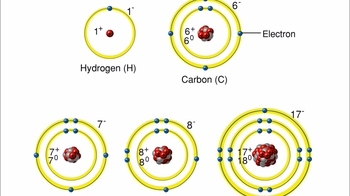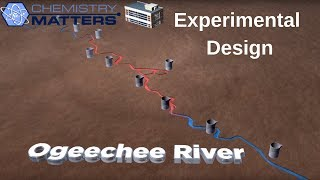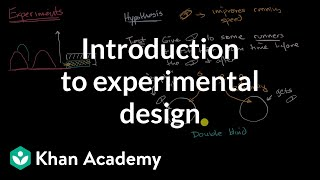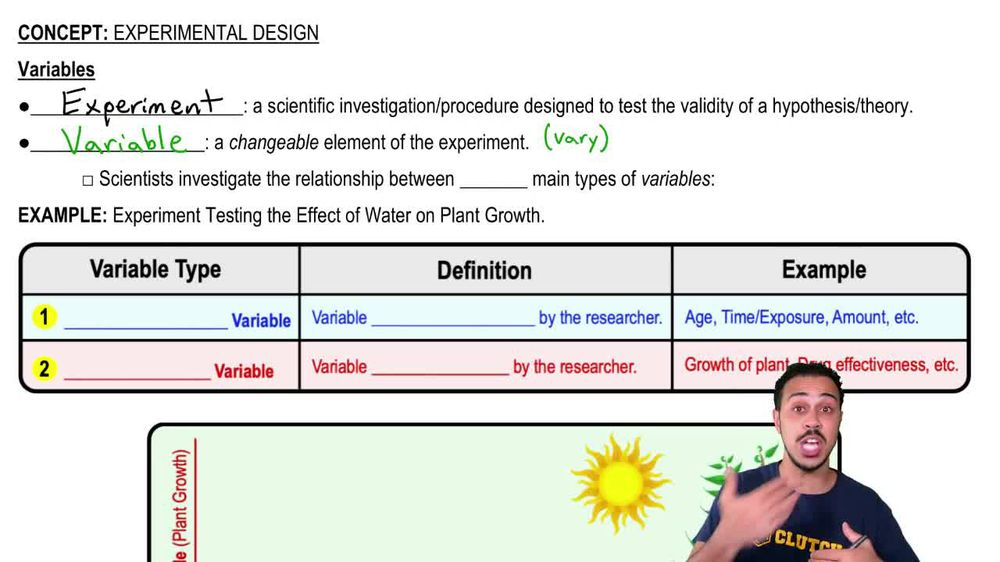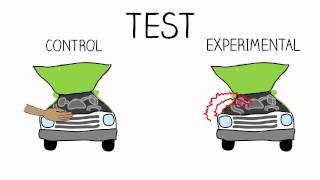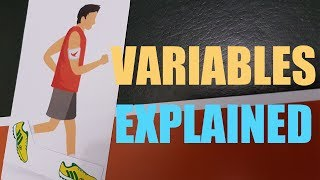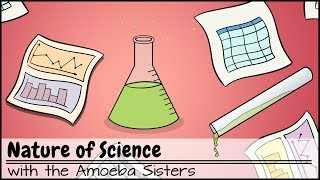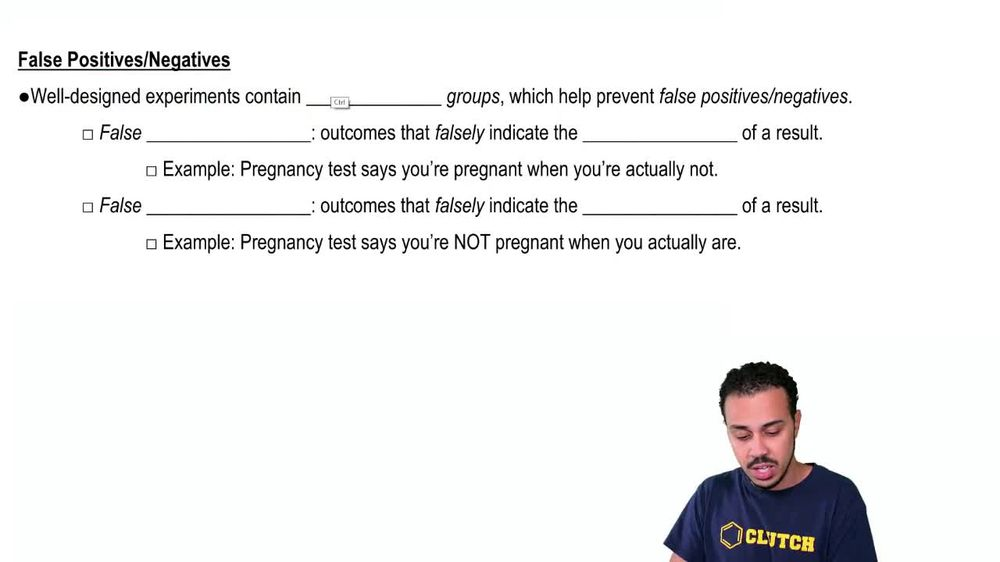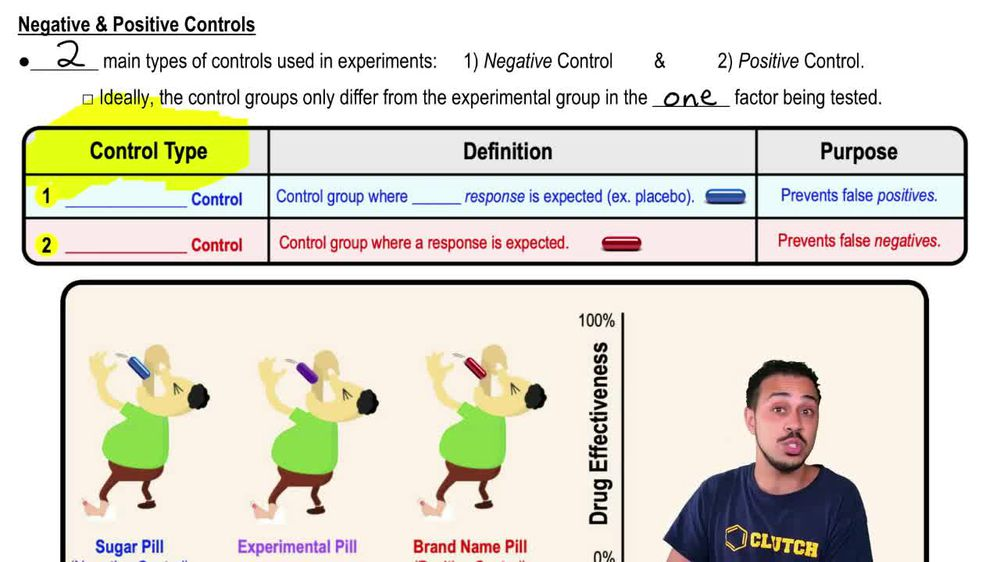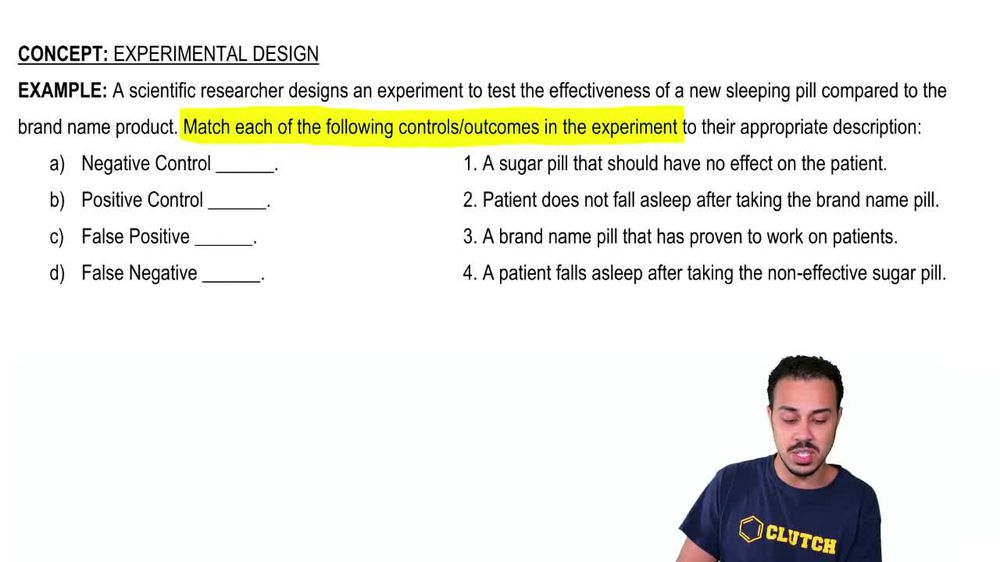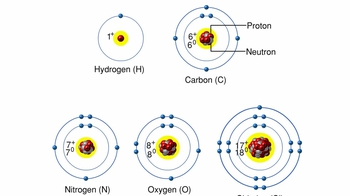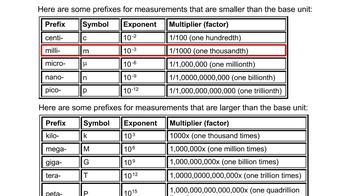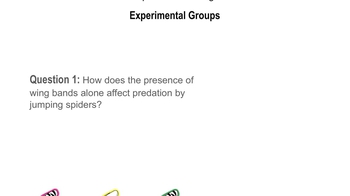Start typing, then use the up and down arrows to select an option from the list.
1. 1. Introduction to Biology2. Experimental Design# Variables

by Jason Amores Sumpter
269 views
4
4
in this video, we're going to talk a little bit about experimental design as it applies to variables of an experiment. And so first we need to define what an experiment is. And so an experiment is defined as a scientific investigation or procedure that's designed to test the validity of a hypothesis or theory. Now a variable. On the other hand, as its name implies here with the root here of the word very, which means things that are going to change. Variables are changeable elements of the experiment, so they're going to change throughout the experiment. Now scientists will investigate the relationship between two main types of variables and an experiment and notice down below. In this table right here, we're going to introduce both of these types of variables, and so the very first variable here is going to be the independent. Variable on the second variable is going to be the dependent variable. Now the independent variable eyes going to be defined as the variable that is controlled or modified by the researcher. And so, for example, over here in this column, we have a few examples that could be independent variables. For instance, the age group of the people used in the experiment can be controlled or modified by the researcher. Maybe the researcher decides to test on the elderly group of people. Maybe they decided to test on the middle age group of people. Or maybe they decide toe test on ah, younger group of people. So that's something that they can control or modify, so that could be an example of an independent variable. Now, another thing that the researcher could control or modify is the amount of time that someone or something is exposed to something. Uh, they could decide to expose them to something for a long period of time, or they could decide to expose them to something for a short period of time so the time or exposure can be controlled or modified by the researcher, and so this could be an example of an independent variable. And then, of course, the amount of, say, a chemical or something like that that the researcher decides to use is something that they can also control or modify. And so once again, these are just a few examples of things that could be independent variables because they can be controlled or modified by the researcher Now, on the other hand, when it comes to the dependent variable, they cannot be controlled or modified by the researcher. So instead, this is going to be defined as the variable that is measured or investigated by the researcher. So since they can't directly control it or modify it, what they do is instead they measure it to see how it changes throughout the experiment. So once again, over here in this column, we have some examples of what could be the dependent variable. It will change on an experiment by experiment basis, but something like the growth of the plant would be an example of a dependent variable. The researcher cannot directly control the growth of the plant, so instead, what they do is they measure the growth of the plant to see how it changes over time and something else could be like the drug effectiveness or the effectiveness of a drug. The researcher cannot directly control or modify it. So instead, what they do is they measure or investigate the effectiveness of the drug and so down below. What we have is an image of a specific experiment that is testing the effect of water on plant growth. And so when we take a look down below at this image, which will notice is we're showing you a graph over here and what you should note about this graph is that on the X axis here, the horizontal axis. Uh, normally, the scientists are going to put the independent variable, which is once again, uh, the variable that is controlled or modified by the researcher. Now, in this particular experiment of testing the effect of water on plant growth, what the researcher has control over is the amount of water that they use to water the plants. And so once again, you can see that amount can be the independent variable. And it is here in this particular example now on the Y axis, which is this vertical axis normally the dependent variable. It's gonna go here, which is the variable that's being measured by the researcher. And so, for this particular example of testing the effect of water on plant growth, the plant growth is going to be the dependent variable. And so what you can see is over here what we have eyes A little set up of the experiment notice that we have two identical plants on. Really. The only difference is that this plant is given little h 20 little water by the researcher. Whereas this plant over here is given Ah, high amount of H 20 or a lot of water by the researcher. And so what you'll see is that, uh the less water the plant receives, the less growth, the less plant growth we get. Whereas the mawr water we give the plant the mawr plant growth we get. And so what you might see is that when the amount of water is really, really low, which would be over here on this axis, we might expect to get very, very little growth. So we could put some, uh, data point over here. However, the mawr water we add, which would be over here on the x axis, uh, the mortgage growth we would expect to get. So we might expect to get a data point somewhere up here with the more water we get. And so you might expect to see a trend that looks something like this with this experiment on DSO. This is just some expected data here. It's not really data. It's just fake data for this example. But the idea here is to really distinguish between the two types of variables the independent variable, which the researcher controls and modifies, and the dependent variable, which the researcher measures. And so this here concludes, our introduction to the different types of variables, and moving forward will be able to get a little bit of practice with this, so I'll see you guys in our next video.